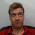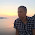## Thursday, April 10, 2014

### On the signs of the order parameters

[updated 7.5.2014]

Until now, only the absolute values of order parameters have been looked at in the nmrlipids project. However, already the first comment to this blog pointed out the relevance of the signs. (I was a little bit sloppy in my reply and said that the signs of the order parameters are correct in Berger; this is not true as mentioned here and dicussed below). It is important to take the sign into account when the order parameters are discussed in relation to the structure of lipid molecules: Two C-H bonds whose order parameters have the same magnitude but different signs are sampling different orientations. Consequently, the correct signs are crucial if force field parameters are fitted to reproduce the correct order parameters, for example, by using the method suggested by Antti Lamberg in this blog. Thus, I think that from now on we have to start looking also at the signs of the order parameters, not just their magnitude.

Note that in the extensive comparison of the headgroup and glycerol order parameters in fully hydrated lipid bilayers we have gathered for the nmrlipids project only the magnitudes are discussed. I think that (for this project) we do not need to reconsider all these results with the sign, since if the absolute value is not in good agreement with experiment, then the value with sign will not be either.

The signs in experiments: tail region
It can be easily seen from the definition of the C-H order parameter
$$S_{CH}=\frac{3}{2}\langle \cos^2 \theta \rangle - \frac{1}{2}$$
that the possible values are  $$-\frac{1}{2} < S_{CH} < +1$$. More specifically
$$-\frac{1}{2} < S_{CH} < 0 \quad \mathrm{, when }\quad 0<\langle\cos^2 \theta\ \rangle < \frac{1}{3},$$
and
$$0 < S_{CH} < 1 \quad \mathrm{, when }\quad \frac{1}{3} < \langle\cos^2 \theta\ \rangle < 1.$$
In older publications that used $$^2$$H NMR measurements, only the absolute values of the order parameters (or quadrupolar splittings) were discussed since the sign was not measurable. For the tail C-H vectors, however, the sign was believed to be negative because $$\theta$$ was expected to fluctuate around 90$$^{o}$$ leading to negative order parameters [Seelig (1977)]. This was later confirmed by using $$^{13}$$C NMR measurements [Hong et al. (1995)]. In simulations, the acyl chain order parameters are typically in good agreement with experiments, including the negative sign (see e.g. Ollila et al (2007)).

The signs in experiments: headgroup and glycerol region
The signs of the ¹³C-H order parameters have been measured by at least two different techniques and groups: Hong et al. first measured eggPC [Hong et al. (1995)] and then DMPC [Hong et al. (1995)]; later Gross et al. used a different NMR technique to measure DMPC [Gross et al. (1997)]. These experiments are in good agreement with one another and report negative order parameters for almost all the segments, only $$\alpha$$ and $$\gamma$$ are positive.

The signs of the order parameters are also listed in Table 2 of the paper by Aussenac et al. (2003), who conducted ²H NMR measurements for bicelles containing DMPC. However, the signs in the table differ from those reported by Hong et al. (1995), Hong et al. (1995) and Gross et al. (1997): Aussenac et al. give positive signs for one of the hydrogens in g1, g3 and C2 in the acyl chain. This was also noted by Högberg et al. when comparing the results to MD simulations. (The paper by Aussenac et al. is slightly confusing to me since I cannot find any discussion concerning the signs or any description of how they were measured; I would warmly welcome some help from an NMR expert to understand how the signs in the paper by Aussenac have been deduced.)
Update: Patrick Fuchs pointed out that Aussenac et al. have not actually measured the signs but used a model to extract the signs. This confirms the original conclusion that the signs from Hong et al. (1995)  and Gross et al. (1997) should be used.

In conclusion, it seems reasonable to assume that the signs measured with ¹³C NMR methods by Hong et al. (1995)  and Gross et al. (1997) are correct. Furthermore, it seems that the signs [Hong et al. (1995), Hong et al. (1995), Gross et al. (1997)] and the absolute values [Gally et al. (1981), Ferreira et al. (2013)] are practically unaffected by the acyl tail contents of the bilayers. Thus, I will also assume that the signs for DPPC and POPC bilayers are the same as measured for eggPC and DMPC. To sum up: the signs for almost all the carbons are negative, only $$\alpha$$ and $$\gamma$$ are positive.

The signs in experiments: varying conditions
Typically when the response of order parameters to varying conditions (ions, dehydration and cholesterol) is measured, only the absolute values are reported. Where clear responses are observed, like for multivalent ions and dehydration, the experiments are done by gradually changing the conditions. The responses of the magnitudes to these changes are systematic, see Figs. 1-3 in the Accuracy post. Thus, it is reasonable to assume that also the signs are not suddenly changing. However, it seems that the sign of the $$\alpha$$ carbon order parameter does change in response to a large amount of bound charge, such as multivalent ions. In this case, the absolute value of the order parameter first decreases to zero and then starts to increase again [Altenbach et al. (1984), Seelig et al. (1987)]; the behaviour of the spectra has been nicely illustrated by Altenbach et al., see Fig. 1.Figure 1. Quadrupolar splitting $$\Delta \nu_Q$$ as a function of CaCl$$_2$$ concentration. As discussed in the Accuracy post, this is related to the order parameter as $$S_{CD}=0.00784 \times \Delta \nu_Q$$. We know nowadays that the order parameter of $$\alpha$$ in the absense of CaCl$$_2$$ is positive [Hong et al. (1995), Hong et al. (1995) and Gross et al. (1997)]. Thus, the most obvious interpertation for the result is that the $$\alpha$$ order parameter decreases to zero when CaCl$$_2$$ concentration reaches 2.0M, and above these concentrations becomes increasingly negative with further addition of CaCl$$_2$$. Reprinted with permission from Altenbach and Seelig, Biochemistry, 23, 3913 (1984). Copyright 1984 American Chemical Society.

Comparison to simulations of fully hydrated pure PC bilayers
The measured order parameters for the fully hydrated POPC bilayer from Ferreira et al. (2013)  with the signs determined by Hong et al. (1995) and Gross et al. (1997) are shown in Fig 2 together with the simulation results for the top 3 force fields  (CHARMM36, GAFFlipids and MacRog, see the lipid force field comparison post), the Berger force field, and the modified Berger force field by Antti Lamberg.Figure 2. Order parameters with signs from experiments and simulations. Note that the y-axis is inverted to make the figure more easily comparable with the plots with the absolute value of order parameter. The magnitudes for the experimental results are taken from Ferreira et al. (2013) and the signs from Hong et al. (1995) , Hong et al. (1995) and Gross et al. (1997)
The signs from all the simulations in Fig. 2 are correct for the carbons $$\alpha$$, g3 and g2. The signs for $$\beta$$, however, are positive in simulations with MacRog, Berger and Lamberg models while experiments give negative values. In the Berger and Lamberg models also the sign of the larger order parameter for g1 is positive while negative value is measured in experiments. This means that the order parameter for the $$\beta$$ carbon in the MacRog model is not as good as it seems when looking only at absolute values. The same applies for the $$\beta$$ and g1 carbons in the Berger and Lamberg models.

1.I have rechecked all the data I have for signs (mainly interested in the 128 simulations with randomized parameters around Berger), and the results are in. http://amlrg.kapsi.fi/nmr/signs.png (beta1, beta2, alpha1, etc on the x-axis), so the first two and the last 5 should be negative accoring to Samuli's post.

So it would seem that it is difficult to change the signs of Berger. I will try to do this at a later date, but the figure does not look particularly promising.

1.But there are some parameters which gives correct signs for g1, right? Especially the blue point seems pretty good. Maybe you could take these parameters as a reference and try a new fit?

2.Yes, there clearly are, and I will be looking into this later. But the fact that one of the beta parameters is never negative, and that g1 is very rarely negative (and not much when it is) is slightly discouraging. Of course, the dihedral parameters are drawn from a Gaussian distribution centered at the original Berger parameters, so it is naturally possible that by doing a couple of iterations one might find parameters that provide a better fit to the experimental data. I do, however, think that to provide a better fit one might need to start tuning the partial charges, too.

3.It might be reasonable to consider tuning the 1-4 interactions as well.

2.While writing this post I realized that in all the force fields where the response of the beta carbon to the dehydration and ions was opposite to the experiments (i.e. MacRog, Orange, Berger and Lamberg force fields), also the sign of the beta carbon order parameter was different than in experiments. I think that this might be the origin of the seemingly disagreement between experiments and simulations, since only the absolute values were compared in the responses. I am currently taking a more careful look into the issue but I think that the beta carbon order parameters are actually getting more positive or more negative due to the changing conditions in all simulations and experiments. If the sign of the value is different these might look qualitatively different responses if only the absolute values are plotted. Thus I think that the qualitative responses of the studied force fields might actually be correct, opposite to the previous discussions in this project.

I will write a new post with more careful discussion. However, for the post I need the MacRog results for the dehydration and ion responses with the signs. Could somebody put those as a reply for this comment?

1.The signs are as with full hydration except for two cases:

For increasing salt concentration, beta signs decrease from positive to negative.:
0: +,+
100: +,–
200: –,–
300: –,–
400: –,–

In case of dehydration, g1 goes as follows:
5: –,–
10: –,–
15: +,–
20: +,–
25 +,–
50 +,–

The smaller value is naturally the one which changes sign.

In these two cases the absolute values are small and therefore the changes in sign do not correspond to large changes in the values.